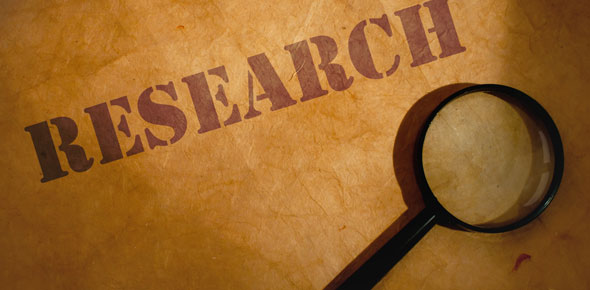# Research Methods

35 Questions | Total Attempts: 87SettingsRelated Topics
• 1.
__________________ is any characteristic whose values can change (what researchers want to study)
• 2.
__________________ __________________ is a definition that translates the variable we want to assess into a specific procedure or measurement
• 3.
The variable being measured/recorded during an experiment
• A.

Dependent

• B.

Independent

• 4.
The variable the experimenter manipulates as a basis for making predictions about the dependent variable
• A.

Dependent

• B.

Independent

• 5.
The __________________ is the entire group about which the investigator wants to draw conclusions
• 6.
The __________________ is the subset of the population that the investigator studies in order to learn about the population at large
• 7.
__________________ __________________ is where every member of the population has an equal chance of being selected for the sample
• 8.
__________________ __________________ __________________ is a strategy of deliberately seeking out the unusual or extreme cases
• 9.
__________________ __________________ is a method of study where the researcher observes and records behavior in organism's natural environment
• 10.
A method that allows researchers to collect a lot of data in a short amount of time
• A.

Case Study

• B.

Survey

• C.

Correlational Studies

• D.

Experiment

• 11.
__________________ __________________ is the degree to which a study's participants, stimuli, and procedures adequately reflect the world as it actually is
• 12.
__________________ __________________ is the characteristic of a study that allows us to conclude that the manipulation of the independent variable caused the observed changes in the dependent variable
• 13.
__________________: the degree to which we're measuring what we intend to measure
• 14.
__________________: how consistently something is measured
• 15.
The __________________ is the most frequent score
• 16.
The __________________ is the halfway point in a data set
• 17.
The __________________ is the arithmetic average of the score
• 18.
The __________________ is the difference between highest and lowest scores
• 19.
The __________________ __________________ is the average difference between each score and the mean of the data set
• 20.
The __________________ __________________ is a calculation that describes the likelihood that the results of a study happened by chance
• 21.
This is measured by the __________________
• 22.
The __________________ __________________ is a number that expresses correlation strength
• 23.
A __________________ __________________ __________________ is when neither the person collecting the data nor the participant knows the hypothesis
• 24.
__________________ __________________ __________________ is when a study compares the data about each participant in one situation to data about the same participant in another situation
• 25.
__________________ is a statistical technique for combining the results of many studies on a particular topic, even when the studies used different data collection methods